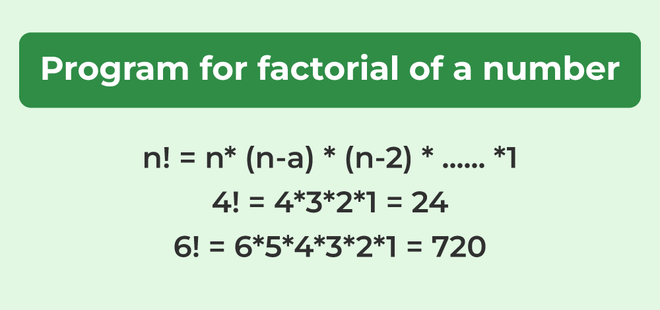# Java Program for factorial of a number

Factorial of a non-negative integer, is multiplication of all integers smaller than or equal to n. In this article, we will learn how to write a program for the factorial of a number in Java.

### Formulae for Factorial

`n! = n * (n-1) * (n-2) * (n-3) * ........ * 1`### Example of Factorial in Java

6! == 6*5*4*3*2*1 = 720.
5! == 5*4*3*2*1 = 120
4! == 4*3*2*1 = 24

## Methods to Find Factorial of a Number

### 1. Iterative Solution for Factorial in Java

Below is the implementation of the above method:

## Java

 `// Java program to find factorial ` `// of given number ` ` `  `// Driver Class ` `class` `Test { ` `    ``// Method to find factorial ` `    ``// of given number ` `    ``static` `int` `factorial(``int` `n) ` `    ``{ ` `        ``int` `res = ``1``, i; ` `        ``for` `(i = ``2``; i <= n; i++) ` `            ``res *= i; ` `        ``return` `res; ` `    ``} ` ` `  `    ``// main method ` `    ``public` `static` `void` `main(String[] args) ` `    ``{ ` `        ``int` `num = ``5``; ` `        ``System.out.println(``"Factorial of "` `+ num + ``" is "` `                           ``+ factorial(``5``)); ` `    ``} ` `}`

Output

```Factorial of 5 is 120
```

#### The complexity of the above method:

Time Complexity: O(n)
Auxiliary Space: O(1)

### 2. Factorial in Java Using Recursive Method

Below is the implementation of the above method:

## Java

 `// Java program to find factorial ` `// of given number ` ` `  `// Driver Class ` `class` `Test { ` `    ``// method to find factorial ` `    ``// of given number ` `    ``static` `int` `factorial(``int` `n) ` `    ``{ ` `        ``if` `(n == ``0``) ` `            ``return` `1``; ` ` `  `        ``return` `n * factorial(n - ``1``); ` `    ``} ` ` `  `    ``// main method ` `    ``public` `static` `void` `main(String[] args) ` `    ``{ ` `        ``int` `num = ``5``; ` `        ``System.out.println(``"Factorial of "` `+ num + ``" is "` `                           ``+ factorial(``5``)); ` `    ``} ` `}`

Output

```Factorial of 5 is 120
```

#### The complexity of the above method:

Time Complexity: O(n)
Auxiliary Space: O(n)

### 3. One-line Solution (Using the Ternary operator)

Below is the implementation of the above method:

## Java

 `// Java program to find factorial ` `// of given number ` ` `  `// Driver Class ` `class` `Factorial { ` `    ``// function to find factorial ` `    ``int` `factorial(``int` `n) ` `    ``{ ` ` `  `        ``// single line to find factorial ` `        ``return` `(n == ``1` `|| n == ``0``) ? ``1` `                                  ``: n * factorial(n - ``1``); ` `    ``} ` ` `  `    ``// main function ` `    ``public` `static` `void` `main(String args[]) ` `    ``{ ` `        ``Factorial obj = ``new` `Factorial(); ` `        ``int` `num = ``5``; ` `        ``System.out.println(``"Factorial of "` `+ num + ``" is "` `                           ``+ obj.factorial(num)); ` `    ``} ` `}`

Output

```Factorial of 5 is 120
```

#### The complexity of the above method:

Time Complexity: O(n)
Auxiliary Space: O(n)

The above solutions cause overflow for small numbers. Please refer factorial of large numbers for a solution that works for large numbers. Please refer complete article on the Program for factorial of a number for more details!

Whether you're preparing for your first job interview or aiming to upskill in this ever-evolving tech landscape, GeeksforGeeks Courses are your key to success. We provide top-quality content at affordable prices, all geared towards accelerating your growth in a time-bound manner. Join the millions we've already empowered, and we're here to do the same for you. Don't miss out - check it out now!

Previous
Next